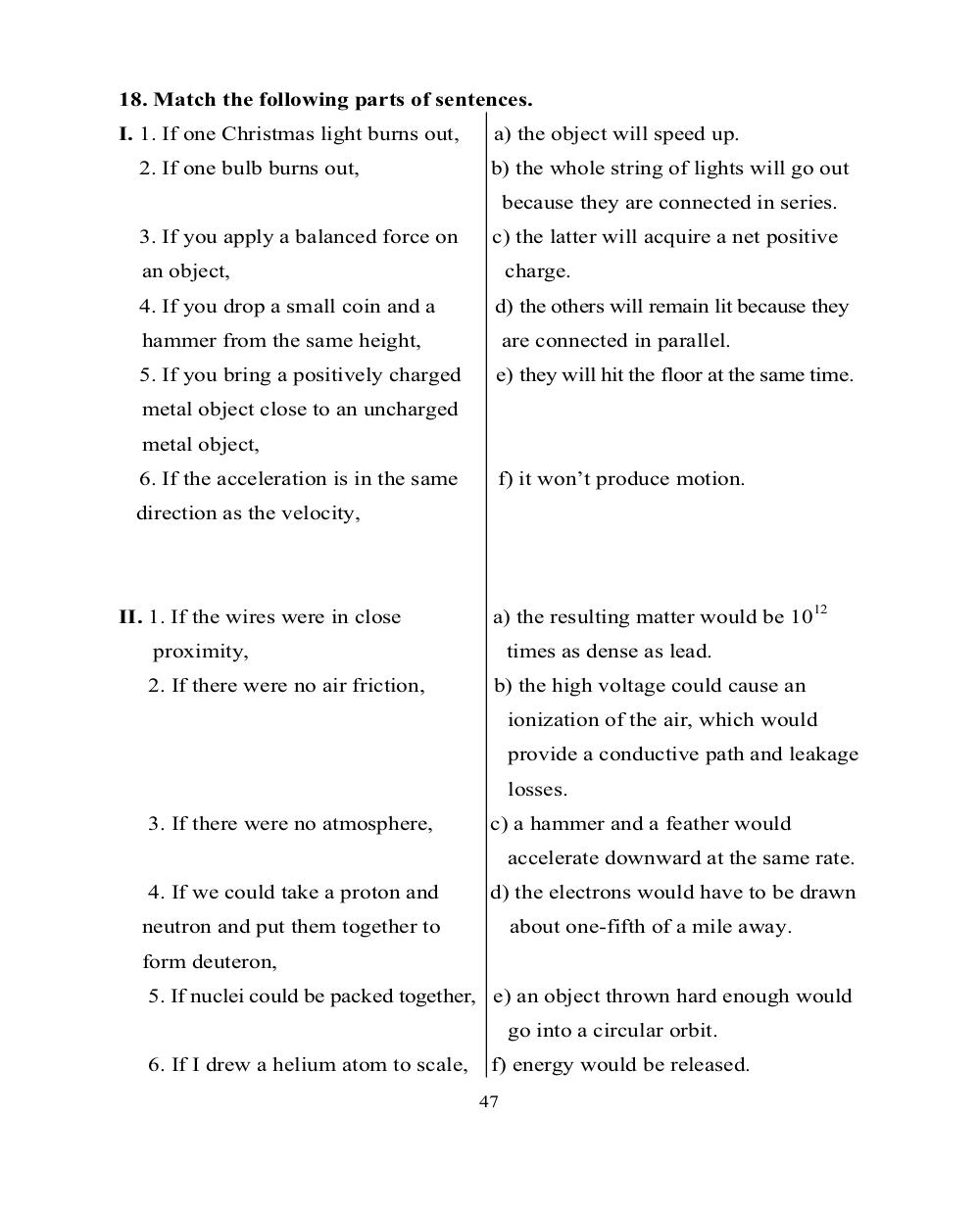# Английский язык. Ч.3 (Units 9,10). Ильичева Н.А - 47 стр.

Составители:

Рубрика:

• ## Иностранный язык18. Match the following parts of sentences.
I. 1. If one Christmas light burns out, ɚ) the object will speed up.
2. If one bulb burns out, b) the whole string of lights will go out
because they are connected in series.
3. If you apply a balanced force on c) the latter will acquire a net positive
an object, charge.
4. If you drop a small coin and a d) the others will remain lit because they
hammer from the same height, are connected in parallel.
5. If you bring a positively charged e) they will hit the floor at the same time.
metal object close to an uncharged
metal object,
6. If the acceleration is in the same f) it won’t produce motion.
direction as the velocity,
II. 1. If the wires were in close a) the resulting matter would be 10
12
proximity, times as dense as lead.
2. If there were no air friction, b) the high voltage could cause an
ionization of the air, which would
provide a conductive path and leakage
losses.
3. If there were no atmosphere, c) a hammer and a feather would
accelerate downward at the same rate.
4. If we could take a proton and d) the electrons would have to be drawn
neutron and put them together to about one-fifth of a mile away.
form deuteron,
5. If nuclei could be packed together, e) an object thrown hard enough would
go into a circular orbit.
6. If I drew a helium atom to scale, f) energy would be released.
4718. Match the following parts of sentences.
I. 1. If one Christmas light burns out,    �) the object will speed up.
2. If one bulb burns out,                b) the whole string of lights will go out
because they are connected in series.
3. If you apply a balanced force on      c) the latter will acquire a net positive
an object,                                   charge.
4. If you drop a small coin and a        d) the others will remain lit because they
hammer from the same height,                 are connected in parallel.
5. If you bring a positively charged     e) they will hit the floor at the same time.
metal object close to an uncharged
metal object,
6. If the acceleration is in the same    f) it won’t produce motion.
direction as the velocity,

II. 1. If the wires were in close          a) the resulting matter would be 1012
proximity,                                 times as dense as lead.
2. If there were no air friction,       b) the high voltage could cause an
ionization of the air, which would
provide a conductive path and leakage
losses.
3. If there were no atmosphere,         c) a hammer and a feather would
accelerate downward at the same rate.
4. If we could take a proton and        d) the electrons would have to be drawn
neutron and put them together to             about one-fifth of a mile away.
form deuteron,
5. If nuclei could be packed together, e) an object thrown hard enough would
go into a circular orbit.
6. If I drew a helium atom to scale, f) energy would be released.
47